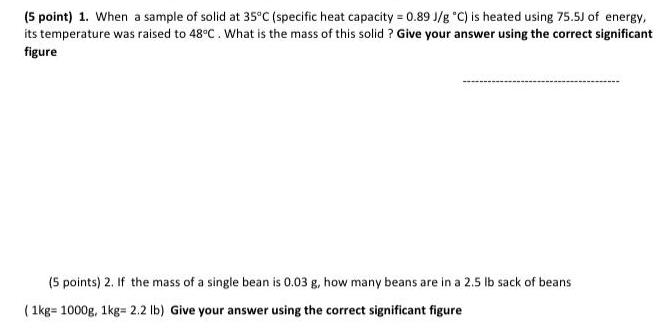Question:

# When a sample of solid at 35°C (specific heat capacity =

Last updated: 8/7/2022When a sample of solid at 35°C (specific heat capacity = 0.89 J/g °C) is heated using 75.5J of energy, its temperature was raised to 48°C. What is the mass of this solid? Give your answer using the correct significant figure If the mass of a single bean is 0.03 g, how many beans are in a 2.5 lb sack of beans (1kg=1000g, 1kg= 2.2 lb) Give your answer using the correct significant figure